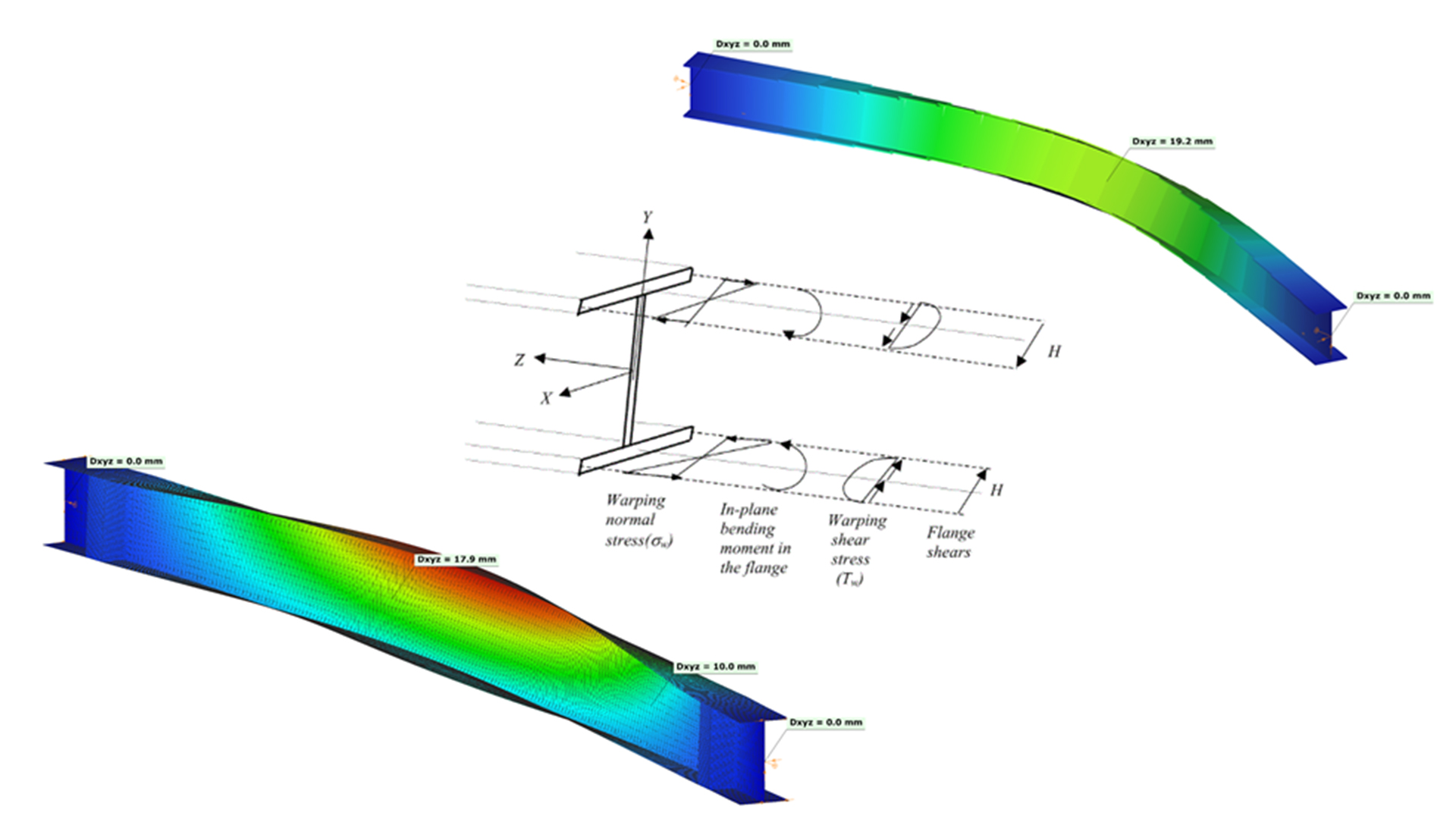EN# Discrete warping restraint

## Theoretical background

According to the beam-column theory, two types of torsional effects exist.

### Saint-Venant torsional component

Some closed thin-walled cross-sections produce only uniform St. Venant torsion if subjected to torsion. For these, only shear stress τoccurs.

### The non-uniform torsional component

Open cross-sections might produce also normal stresses as a result of torsion.[1.]

Warping causes in-plane bending moments in the flanges. From the bending moment arise both shear and normal stresses as it can be seen in Fig. 2 above.

## Discrete warping restraint

The load-bearing capacity of a thin-walled open section against lateral-torsional buckling can be increased by improving the section’s warping stiffness. This can be done by adding additional stiffeners to the section at the right locations, which will reduce the relative rotation between the flanges due to the torsional stiffness of this stiffener. In Consteel, such stiffener can be added to a Superbeam using the special Stiffener tool. Consteel will automatically create a warping support in the position of the stiffener, the stiffness of which is calculated using the formulas below. Of course, warping support can also be defined manually by specifying the correct stiffness value, calculated with the same formulas (see literature ).

The following types of stiffeners can be used:

• Web stiffeners
• T - stiffener
• L - stiffener
• Box stiffener
• Channel –stiffener

The general formula which can be used to determine the stiffness of the discrete warping restraint is the following:

where,

Rω = the stiffness of the discrete warping restraint

G = shear modulus

GIt = the Saint-Venan torsional constant

h = height of the stiffener

### Effect of the different stiffener types

#### Web stiffener

where

b = width of the web stiffener [mm]

t = thickness of the web stiffener [mm]

h = height of the web stiffener [mm]

#### T - stiffener

where

b1 = width of the battens [mm]

t1 = thickness of the battens [mm]

b2 = width of the web stiffener [mm]

t2 = thickness of the web stiffener [mm]

h = height of the web stiffener [mm]

#### L-stiffener

where

b = width of the L-section [mm]

t = thickness of the L-section [mm]

h = height of the L-section [mm]

#### Channel stiffener

where

b1 = width of channel web [mm]

t1 = thickness of channel web [mm]

b2 = width of channel flange [mm]

t2 = thickness of channel flange [mm]

h = height of the web stiffener [mm]

## Numerical example

The following example will show the increase of the lateral-torsional buckling resistance of a simple supported structural beam strengthened with a box stiffeners. The effect of such additional plates can be clearly visible when shell finite elements are used.

### Shell model

Fig. 7 shows a simple fork supported structural member with welded cross-section modeled with shell finite elements and subjected to a uniform load along the member length acting at the level of the top flange.

Table 1. and Table 2. contain the geometric parameters and material properties of the double symmetric I section. The total length of the beam member is 5000 mm, the eccentricity of the line load is 150 mm in direction z.

#### Box stiffener

The box stiffeners are located near the supports as can be seen in Fig. 8. Table 3. contains the geometric parameters of the box stiffeners.

### 7DOF beam model

The same effect in a model using 7DOF beam finite elements can be obtained when discrete warping spring supports are defined at the location of the box stiffeners.

#### Discrete warping stiffness calculated by hand

The warping spring stiffness corresponding to the box stiffeners can be calculated as shown in the previous chapter [2.1.4]. The formula is the following:

## Results

Lateral torsional buckling shapes can be seen in the following pictures (Fig. 10 and Fig 11.). The obtained elastic critical buckling factor with the 7DOF beam model and with the shell model with and without the box stiffeners are compared in Table 4. The results show good agreement. The lateral-torsional buckling resistances of the original and strengthened structural members are calculated using EN 1993-1-1 and shown in Table 5.